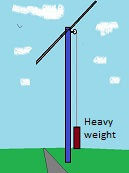Fusion energy, perhaps just dreaming..

Energy storage for wind turbines

Just an idea

A common (big) wind turbine has a power of 5 MW.  ( see http://en.wikipedia.org/wiki/Wind_turbine  )

During one day, 24 hrs, it produces: (24 hrs  = 60 s.60 m.24 hrs)

5.106 . 60.60.24 J = 4,32.1011 J

Could we storage this energy by lifting up a weight?  (Similar as a weight driven clock..)The potential energy Ɛ of a weight (mass) m kg  at h meters above the surface:

Ɛ  = m.g.h     ( g = gravitational acceleration = 9,8 m/s2 )

The energy of the wind turbine produced during one day:

4,32.1011 J = m.9,8.h

Suppose we lift the weight 10 m, then we need a weight of:

m = 4,32.1011 J / 98 4,4 109 kg  = 4,4. 106  ton

The energy of the wind turbine produced during two weeks stored in a weight at 10 m height:

m =  4,4. 106  ton  . 14 = 6,2 . 107 ton

The specific weight of concrete is about 2,3 mt/m3

If the weight is a cubic block of concrete, it should have the dimensions:

6,2 . 107 /  2,3 = 2,7. 107 m3     300 m x 300 m x 300 m     or  =>  1,6 km x 1,6 km x 10 m

A little bit impossible...

But suppose we have a hole with a depth of 400 mtr.

The weight to store the energy of the wind turbine of one day:

4,32.1011 J = m.9,8.400  => m=  1,1.105 ton   concrete block = 4,8 . 10m3 =>  for example  36x36x36 mtr

With water it is more easily,  see:  http://en.wikipedia.org/wiki/Pumped-storage_hydroelectricity

21 June  2014      by  Rinze Joustra        www.valgetal.com